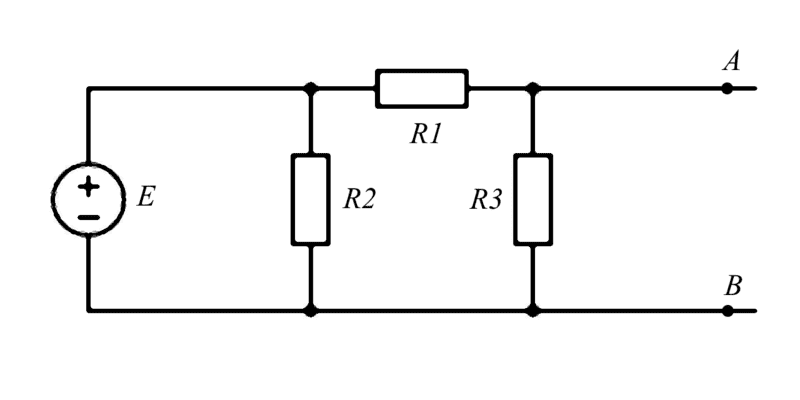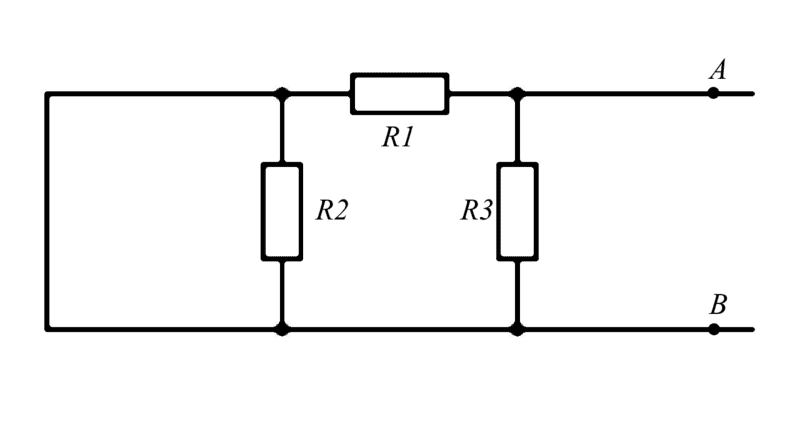# Basic current question

• archaic

#### archaic

Moved from a technical forum, no template.
Hey guys, in the picture below, the current flowing through R1 is the same flowing through R3 right? And hence we have R1 and R3 in series?In this second picture R2 is going to cancel out because apparently that's what happens when you have a branch containing components parallel with a wire, why is that?#### Attachments

Last edited:

Hey guys, in the picture below, the current flowing through R1 is the same flowing through R3 right? And hence we have R1 and R3 in series?
Right, assuming that there are no circuit elements beyond points A and B.
In this second picture R2 is going to cancel out because apparently that's what happens when you have a branch parallel with a wire, why is that?
That's because R2 is shorted, i.e. the potential difference across it zero (think of the straight wire as an equipotential). Ohm's Law says V = IR. Here, V = 0 therefore I = 0 and R2 draws no current, assuming that there is a potential difference between A and B.

Please use the homework forum, and please fill out the template provided when you create a homework thread.

In the top diagram, provided that A-B is open circuit (no current), then the answer is yes. You are correct.

In this second picture R2 is going to cancel out because apparently that's what happens when you have a branch parallel with a wire, why is that?
How much current flows through R2 in the second picture?

Right, assuming that there are no circuit elements beyond points A and B.
Yes, it's an open circuit, but this is part of a Thevenin's theorem exercise so we're assuming there's an open circuit voltage at the terminals A and B.

That's because R2 is shorted, i.e. the potential difference across it zero (think of the straight wire as an equipotential). Ohm's Law says V = IR. Here, V = 0 therefore I = 0 and R2 draws no current, assuming that there is a potential difference between A and B.
How much current flows through R2 in the second picture?
Well as kuruman said the current is 0, the explanation we were given is "the current takes the less difficult path" but how would it know which one it is?

Well as kuruman said the current is 0, the explanation we were given is "the current takes the less difficult path" but how would it know which one it is?
Well, how much resistance is there in a short circuit?

Well, how much resistance is there in a short circuit?
0 of course.

0 of course.
And you are having a hard time figuring out whether this is less than R2?

And you are having a hard time figuring out whether this is less than R2?
No but I want to know how electrons figure it out before going through the wire.

Change it a bit. Think of the wire in parallel with R2 as having resistance R4. Then calculate the equation for R2 in parallel with R4. Then set R4=0, how much is resistance is R2 in parallel with R4?

•archaic